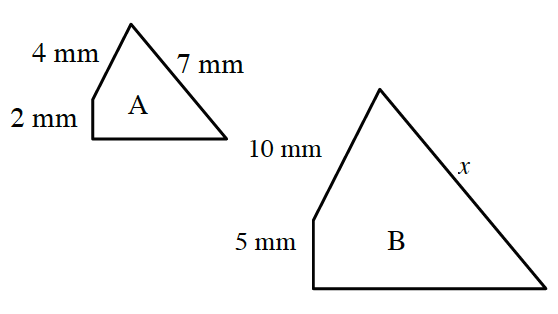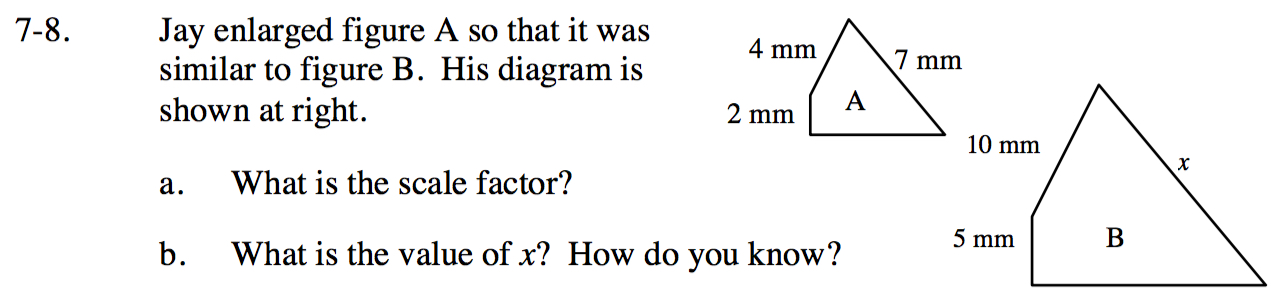### Home > CC4 > Chapter 7 > Lesson 7.1.1 > Problem7-8

7-8.
1.Jay enlarged figure A so that it was similar to figure B. His diagram is shown at right. Homework Help ✎

1. What is the scale factor?

2. What is the value of x? How do you know?Read Math Notes box in Lesson 7.1.1 to determine how to write a scale factor.
Remember a scale factor is a ratio (fraction).

Which side of figure A corresponds to side x in figure B?
Multiply that side length by the scale factor you found in part (a)..